• python 聚类分析实战案例:K-means算法(原理源码)
2020-11-29 06:58:17

K-means算法：

关于步骤：参考之前的博客

关于代码与数据：暂时整理代码如下：后期会附上github地址，上传原始数据与代码完整版，

各种聚类算法的对比：参考连接

Kmeans算法的缺陷

1.聚类中心的个数K 需要事先给定，但在实际中这个 K 值的选定是非常难以估计的，很多时候，事先并不知道给定的数据集应该分成多少个类别才最合适

2.Kmeans需要人为地确定初始聚类中心，不同的初始聚类中心可能导致完全不同的聚类结果。#!usr/bin/env python

#_*_ coding:utf-8 _*_

import random

import math

'''

'''

def importData():

f = lambda name,b,d: [name, float(b), float(d)]

with open('birth-death-rates.csv', 'r') as inputFile:

return [f(*line.strip().split('\t')) for line in inputFile]

写入文件类型

#2. calculate Distancedef euclideanDistance(x,y):

return math.sqrt(sum([(a-b)**2 for (a,b) in zip(x,y)]))

#L=points,

def partition(points, k, means, d=euclideanDistance):

# print('means={}'.format(means))

thePartition = [[] for _ in means] # list of k empty lists

indices = range(k)

# print('indices={}'.format(indices))

for x in points:

#index为indices索引，调用d函数，计算每个值与聚类中心的距离，将其分类

closestIndex = min(indices, key=lambda index: d(x, means[index]))#实现X与每个Y直接的求解：key=lambda index: d(x, means[index])

thePartition[closestIndex].append(x)

return thePartition#3.寻找收敛点

def mean(points):

''' assume the entries of the list of points are tuples;

e.g. (3,4) or (6,3,1). '''

n = len(points)

# print(tuple(float(sum(x)) / n for x in zip(*points))) #*points将【[1，2]，[2，3]】分割出来【1，2】

return tuple(float(sum(x)) / n for x in zip(*points)) #将最开始的[[4, 1], [1, 5]] 经过处理变成[（4, 1）,（1, 5）]

def kMeans(points, k, initialMeans, d=euclideanDistance):

oldPartition = []

newPartition = partition(points, k, initialMeans, d)

while oldPartition != newPartition:

oldPartition = newPartition

newMeans = [mean(S) for S in oldPartition]

newPartition = partition(points, k, newMeans, d)

return newPartition

#0.函数调用初始中心点if __name__ == "__main__":

L = [x[1:] for x in importData()] # remove names

# print (str(L).replace('[','{').replace(']', '}'))

import matplotlib.pyplot as plt

'''

plt.scatter(*zip(*L))

plt.show()

'''

import random

k = 3

partition = kMeans(L, k, random.sample(L, k)) #L是集合，K分类个数，random.sample(L, k)中心点

plt.scatter(*zip(*partition), c='b')#[[],[],[]]

plt.scatter(*zip(*partition), c='r')

plt.scatter(*zip(*partition), c='g')

plt.show()

更多相关内容
• Python聚类分析，是无监督的机器学习中的一种！
• 基于Python聚类分析以及应用，Python中聚类方法的详细解释，以及一些应用
• python 文本聚类分析案例说明摘要1、结巴分词2、去除停用词3、生成tfidf矩阵4、K-means聚类5、获取主题词 / 主题词团 说明 实验要求：对若干条文本进行聚类分析，最终得到几个主题词团。 实验思路：将数据进行预处理...
• scipy.cluster是scipy下的一个做聚类的package, 共包含了两类聚类方法: 1. 矢量量化(scipy.cluster.vq):支持vector quantization 和 k-means 聚类方法 2. 层次聚类(scipy.cluster.hierarchy):支持hierarchical ...

scipy.cluster是scipy下的一个做聚类的package, 共包含了两类聚类方法: 1. 矢量量化(scipy.cluster.vq):支持vector quantization 和 k-means 聚类方法 2. 层次聚类(scipy.cluster.hierarchy):支持hierarchical clustering 和 agglomerative clustering(凝聚聚类)

聚类方法实现:k-means和hierarchical clustering.

###cluster.py#导入相应的包import scipy

import scipy.cluster.hierarchy as sch

from scipy.cluster.vq import vq,kmeans,whiten

import numpy as np

import matplotlib.pylab as plt#生成待聚类的数据点,这里生成了20个点,每个点4维:points=scipy.randn(20,4)

#1. 层次聚类#生成点与点之间的距离矩阵,这里用的欧氏距离:disMat = sch.distance.pdist(points,'euclidean')

#将层级聚类结果以树状图表示出来并保存为plot_dendrogram.pngP=sch.dendrogram(Z)

plt.savefig('plot_dendrogram.png')#根据linkage matrix Z得到聚类结果:cluster= sch.fcluster(Z, t=2, criterion='inconsistent')

print "Original cluster by hierarchy clustering:\n",cluster#2. k-means聚类#将原始数据做归一化处理data=whiten(points)#使用kmeans函数进行聚类,输入第一维为数据,第二维为聚类个数k.#有些时候我们可能不知道最终究竟聚成多少类,一个办法是用层次聚类的结果进行初始化.当然也可以直接输入某个数值. #k-means最后输出的结果其实是两维的,第一维是聚类中心,第二维是损失distortion,我们在这里只取第一维,所以最后有个centroid=kmeans(data,max(cluster))

#使用vq函数根据聚类中心对所有数据进行分类,vq的输出也是两维的,表示的是所有数据的labellabel=vq(data,centroid)

print "Final clustering by k-means:\n",label1234567891011121314151617181920212223242526272829303132333435363738

在Terminal中输入:python cluster.py 输出: Original cluster by hierarchy clustering: [4 3 3 1 3 3 2 3 2 3 2 3 3 2 3 1 3 3 2 2] Final clustering by k-means: [1 2 1 3 1 2 0 2 0 0 0 2 1 0 1 3 2 2 0 0] 数值是随机标的,不用看,只需要关注同类的是哪些.可以看出层次聚类的结果和k-means还是有区别的

运行过程中出现了该问题SyntaxError: non-keyword arg after keyword arg

Python中调用函数时，有时会报SyntaxError: non-keyword arg after keyword arg错误。 这通常是因为函数中定义了部分参数的默认值，Python中*arg表示任意多个无名参数，类型为tuple(元组)，**kwargs表示关键字参数，为dict(字典)，因此没有默认值的参数，即*arg 要放在前面，**kwargs 要放在后面，出现这个错误后，可以在有默认值的参数前加上参数名即可。

cluster= sch.fcluster(Z, t=2, 'inconsistent') 出现上述错误1

cluster= sch.fcluster(Z, t=2, criterion='inconsistent')则正常1

补充:一些函数的用法

1.linkage(y, method=’single’, metric=’euclidean’) 共包含3个参数: y是距离矩阵,由pdist得到;method是指计算类间距离的方法,比较常用的有3种: (1)single:最近邻,把类与类间距离最近的作为类间距 (2)complete:最远邻,把类与类间距离最远的作为类间距 (3)average:平均距离,类与类间所有pairs距离的平均

2.fcluster(Z, t, criterion=’inconsistent’, depth=2, R=None, monocrit=None) 第一个参数Z是linkage得到的矩阵,记录了层次聚类的层次信息; t是一个聚类的阈值-“The threshold to apply when forming flat clusters”,在实际中,感觉这个阈值的选取还是蛮重要的.另外,scipy提供了多种实施阈值的方法(criterion):

3.kmeans(obs, k_or_guess, iter=20, thresh=1e-05, check_finite=True) 输入obs是数据矩阵,行代表数据数目,列代表特征维度; k_or_guess表示聚类数目;iter表示循环次数,最终返回损失最小的那一次的聚类中心; 输出有两个,第一个是聚类中心(codebook),第二个是损失distortion,即聚类后各数据点到其聚类中心的距离的加和.

参考页面:http://docs.scipy.org/doc/scipy/reference/generated/scipy.cluster.vq.kmeans.html#scipy.cluster.vq.kmeans12

4.vq(obs, code_book, check_finite=True) 根据聚类中心将所有数据进行分类.obs为数据,code_book则是kmeans产生的聚类中心. 输出同样有两个:第一个是各个数据属于哪一类的label,第二个和kmeans的第二个输出是一样的,都是distortion

参考页面:

http://docs.scipy.org/doc/scipy/reference/generated/scipy.cluster.vq.vq.html#scipy.cluster.vq.vq123

展开全文• 主要介绍了Python聚类算法之凝聚层次聚类的原理与具体使用技巧,具有一定参考借鉴价值,需要的朋友可以参考下
• 聚类分析又称群分析，它是研究（样品或指标）分类问题的一种统计分析方法，同时也是数据挖掘的一个重要算法。 聚类（Cluster）分析是由若干模式（Pattern）组成的，通常，模式是一个度量（Measurement）的向量，或者...
• 文件包含聚类分析的数据集、python kmeans代码、有效性评估、PPT内容展示，适合需要课程报告的同学使用。
• 核心内容：使用python的sklearn的KMeans算法对电商用户进行分类。 包含内容：数据概览、特征构建、k值选择、模型训练、可视化等。

#### 背景

聚类分析在机器学习领域属于无监督学习的一种，能够根据一些特征对样本数据进行分类。使用聚类分析分完的类具有“类中相似，类间区别”的特点。RFM模型是非常常见的分析用户价值的方法，其核心思想是根据用户的最近购买时间、购买频次、花费金额3个特征来对用户进行分群，针对每个群体采取不同的营销手段。k-means是常用的聚类分析算法之一，基于欧氏距离对样本进行分类。k-means算法运行速度快，能够处理的数据量大，且易于理解。但缺点也很明显，就是算法性能有限，在高维上可能不是最佳选项。在当前动辄上亿的数据量来看，k-means算法是比较好的选择了。还有需要提醒的一点是，一定要结合业务使用算法，任何特征都可能拿来聚类，但是聚类的结果呢，能不能很好的解释和指导业务？如果不能，那么这个算法就没有什么意义。本次使用的数据来源于数据不吹牛公众号，这里也是小打一波广告。
本案例将从一个简单的k-means机器学习模型入手，在介绍聚类算法的同时也简单介绍机器学习的常规步骤。

#### 1、数据概览

首先，我们导入数据，并查看前5行。

import pandas as pd
import numpy as np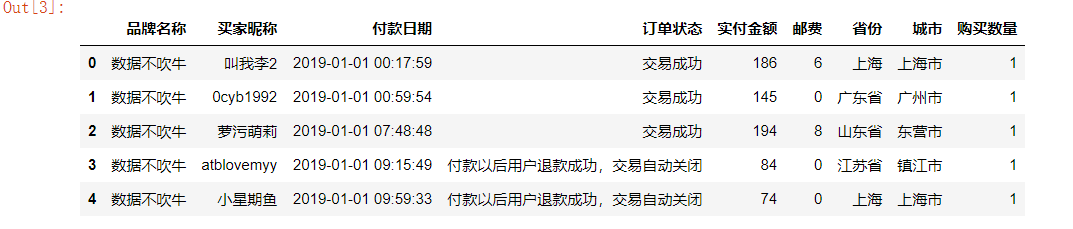总览数据：

df.info()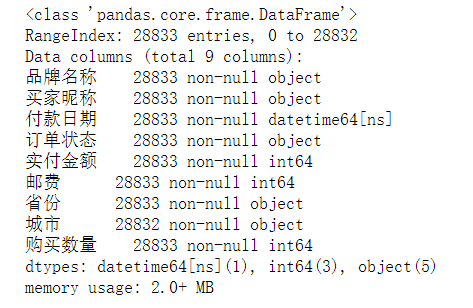数据共有28833行，9列，而且数据非常干净，没有空值，省去了我们处理空值的过程。
根据RFM模型的要求，我们需要的是买家昵称、购买日期、实付金额三个变量。其中购买日期和实付金额用于帮助我们训练模型，买家昵称则让我们确定每个用户所属的类别。

选取交易成功的用户：

df['订单状态'].value_counts()
df = df[df['订单状态'] == '交易成功']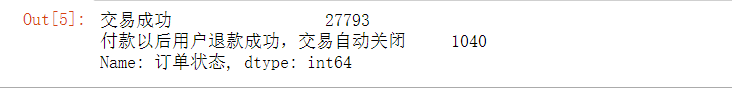选择我们需要的字段：

data = df[['买家昵称', '付款日期',  '实付金额']]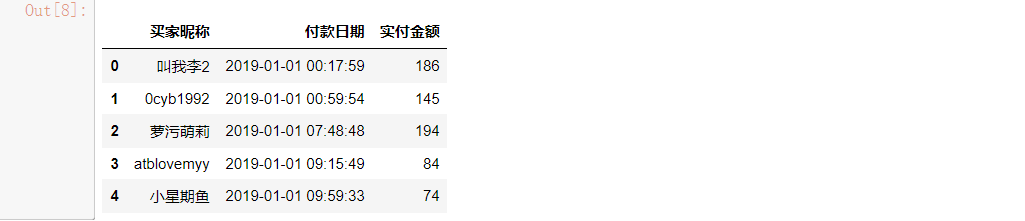有个细节需要注意，订单每一行代表着单个用户的单次购买行为，什么意思呢？如果一个用户在一天内购买了4次，订单表对应记录着4行，而在实际的业务场景中，一个用户在一天内的多次消费行为，应该从整体上看作一次。 ----- 数据不吹牛 小Z

#### 2、特征构建

根据我们的RFM模型，我们需要构建3列数据，这个原始表中是没有直接提供的，需要我们根据原始表的数据来提取。
所以这里需要先提取付款日期数据：

data['paytime'] = pd.to_datetime(data['付款日期'].apply(lambda x:x.date()))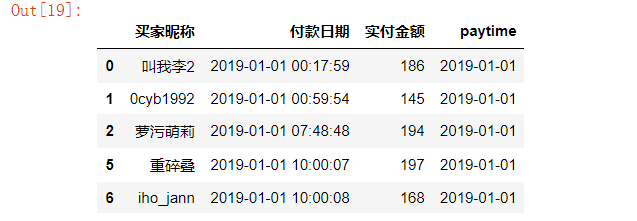根据paytime计算最后一次付款时间距当前的天数（数据引用的背景当前时间是2019-07-01）：

# 提取每个用户最近（最大）的购买日期
data_r = data.groupby('买家昵称')['paytime'].max().reset_index()
# 与当前日期相减，取得最近一次购买距当前的天数。
data_r['recency'] = data_r['paytime'].apply(lambda x:(pd.to_datetime('2019-07-01')-x).days)
# 两个日期相减，得到的数据类型是timedelta类型，要进行数值计算，需要提取出天数数字。
data_r.drop('paytime',axis = 1,inplace = True)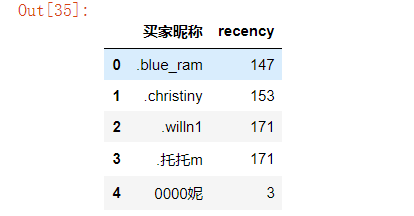提取购买次数数据：

# 分组聚合，得到每个用户发生于不同日期的购买次数
data_f = data.groupby(['买家昵称','paytime'])['付款日期'].count().reset_index()
data_f = data_f.groupby('买家昵称')['paytime'].count().reset_index()
# 修改列名
data_f.rename({'paytime':'frequence'},axis = 1,inplace = True)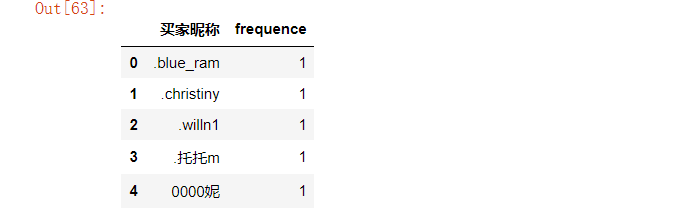提取购买金额数据，这里的金额我们使用每个用户的金额/次：

data_m = data.groupby('买家昵称')['实付金额'].sum().reset_index()
data_m['money'] = data_m['实付金额']/data_f['frequence']
data_m.drop('实付金额',axis = 1,inplace = True)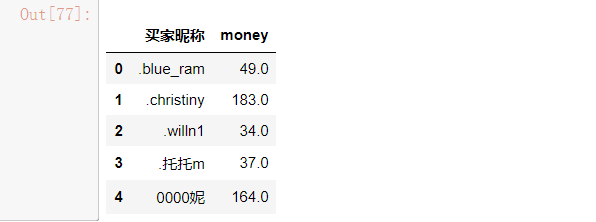所以现在我们已经有了包含recency、frequence、money的3个DataFrame表了，下面合并三个表：

data_rf = pd.merge(data_r,data_f,on = '买家昵称',how = 'inner')
data_rfm = pd.merge(data_rf,data_m, on = '买家昵称',how = 'inner')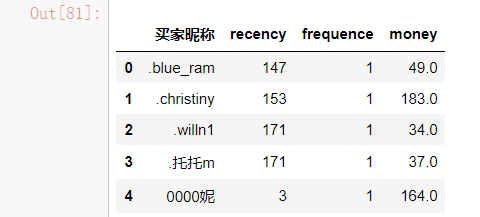#### 3、查看数据分布特征

数据的分布特征会影响算法结果，所以有必要先了解数据的大致分布。

import matplotlib.pyplot as plt
import seaborn as sns
plt.figure(figsize = (6,4))
sns.set(style = 'darkgrid')
sns.countplot(data_rfm['frequence'])sns.distplot(data_rfm['recency'])
plt.title('recency的分布直方图',fontsize = 15)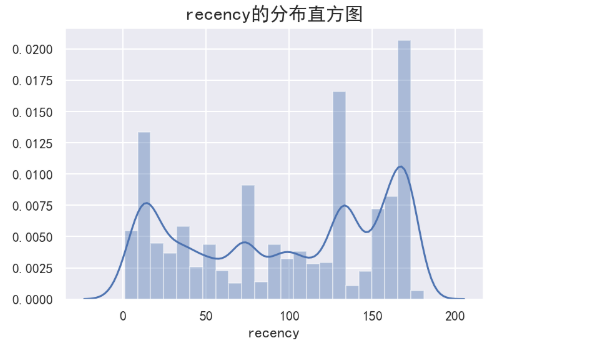sns.distplot(data_rfm['money'],color = 'g')
plt.title('money的分布直方图',fontsize = 15)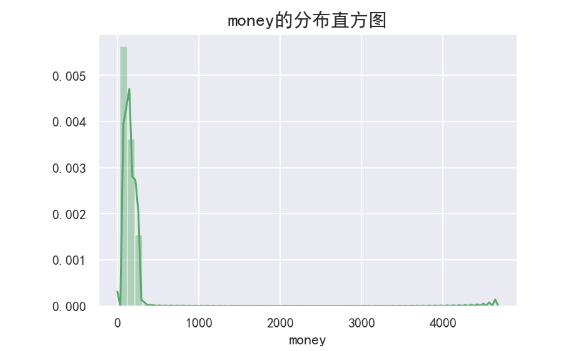#### 4、数据处理及模型构造

首先，对数据进行标准化处理，这是为了消除量纲的影响。

from sklearn import preprocessing
from sklearn.cluster import KMeans
from sklearn import metrics
data_rfm_s = data_rfm.copy()
min_max_scaler = preprocessing.MinMaxScaler()
data_rfm_s = min_max_scaler.fit_transform(data_rfm[['recency','frequence','money']])


K-Means方法有个经常被人诟病的地方，如何选择K值？也就是要把样本分成多少类呢？如果贸然定一个，会太主观，所以还是要用数据说话。一般采用三个指标：

• Calinski-Harabaz Index。通过计算类中各点与类中心的距离平方和来度量类内的紧密度，通过计算各类中心点与数据集中心点距离平方和来度量数据集的分离度，CH指标由分离度与紧密度的比值得到。从而，CH越大代表着类自身越紧密，类与类之间越分散，即更优的聚类结果。
• 轮廓系数。轮廓系数（Silhouette Coefficient），值是介于 [-1,1] ，越趋近于1代表内聚度和分离度都相对较优。也就是尽量选择更大的轮廓系数。
• inertia值。kmeans自带的评价方法，越小说明聚类效果越好。
inertia = []
ch_score = []
ss_score = []
for k in range(2,9):
model = KMeans(n_clusters = k, init = 'k-means++',max_iter = 500)
model.fit(data_rfm_s)
pre = model.predict(data_rfm_s)
ch = metrics.calinski_harabaz_score(data_rfm_s,pre)
ss = metrics.silhouette_score(data_rfm_s,pre)
inertia.append(model.inertia_)
ch_score.append(ch)
ss_score.append(ss)
print(ch_score,ss_score,inertia)


画图可以更加直观看出三个指标的变化：

score = pd.Series([ch_score,ss_score,inertia],index = ['ch_score','ss_score','inertia'])
aa = score.index.tolist()
plt.figure(figsize = (15,6))
j = 1
for i in aa:
plt.subplot(1,3,j)
plt.plot(list(range(2,9)),score[i])
plt.xlabel('k的数目',fontsize = 13)
plt.ylabel(f'{i}值',fontsize = 13)
plt.title(f'{i}值变化趋势',fontsize = 15)
j+=1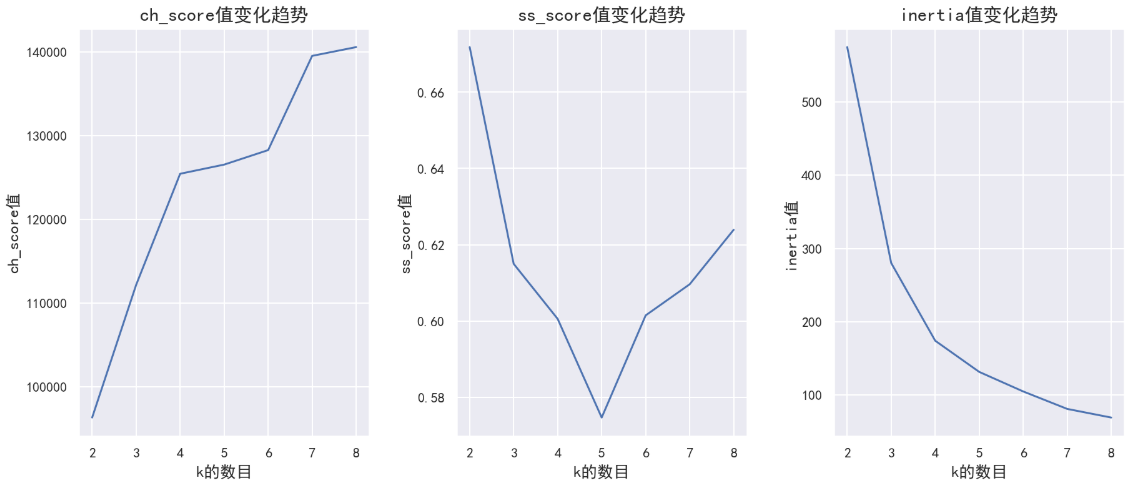根据上图中3个指标综合判断，选择k=4时，各指标变化出现明显的拐点，聚类效果相对较优，所以分成四类比较好。

model = KMeans(n_clusters = 4, init = 'k-means++',max_iter = 500)
model.fit(data_rfm_s)
ppre = model.predict(data_rfm_s)
ppre = pd.DataFrame(ppre)
data = pd.concat([data_rfm,ppre],axis = 1)
data.rename({0:u'cluster'},axis = 1,inplace = True)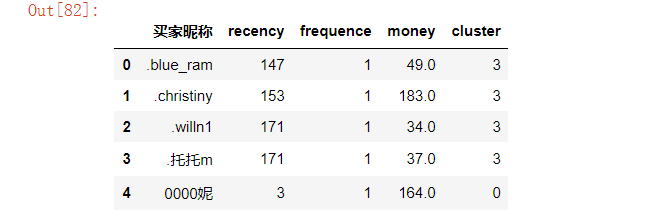可以看出，每个用户都有了对应的类别，并且可以查看每个类别的中心：

labels = model.labels_   # 取得各样本的类别数据
labels = pd.DataFrame(labels,columns = ['类别'])
result = pd.concat([pd.DataFrame(model.cluster_centers_),labels['类别'].value_counts().sort_index()],axis = 1)
result.columns = ['recency','frequence','money','cluster']
result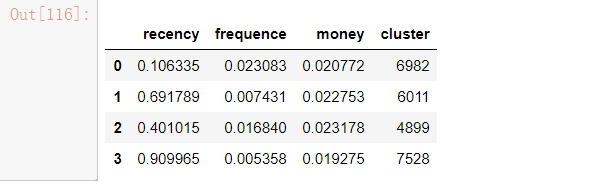cluster=0时的分布状态：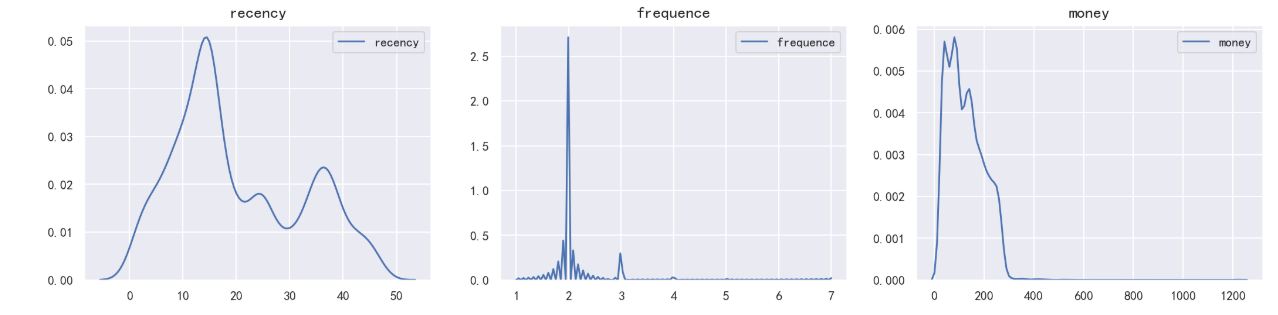cluster=1时的分布状态：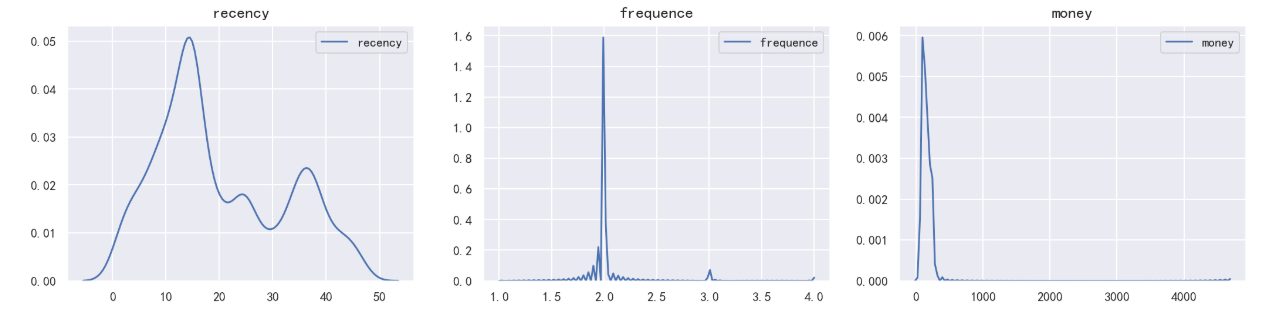cluster=2时的分布状态：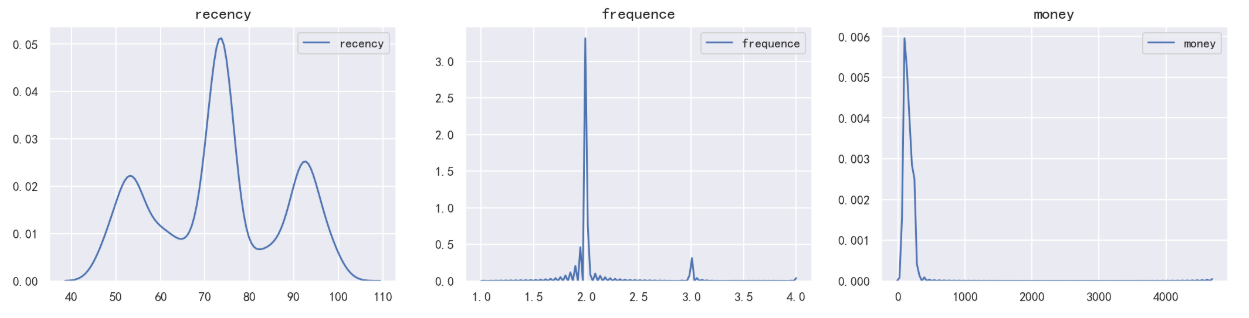cluster=3时的分布状态：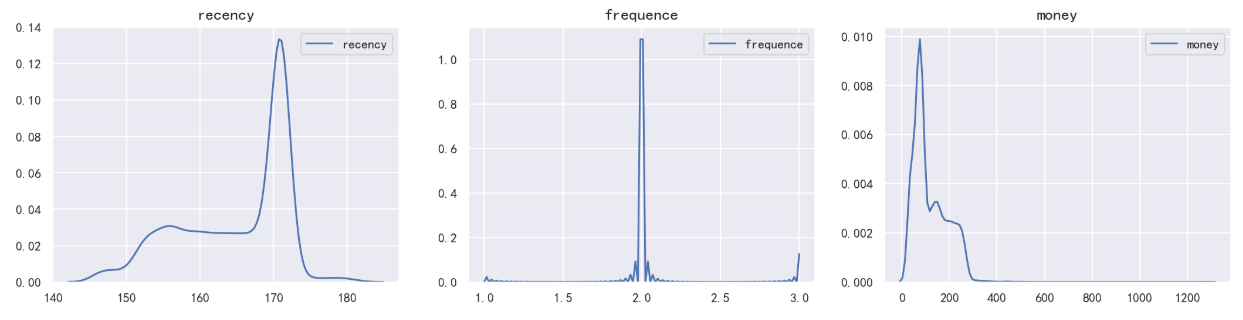展开全文机器学习
• 本文实例为大家分享了Python实现简单层次聚类算法，以及可视化，供大家参考，具体内容如下 基本的算法思路就是：把当前组间距离最小的两组合并成一组。 算法的差异在算法如何确定组件的距离，一般有最大距离，最小...
• 本文实例讲述了Python聚类算法之DBSACN。分享给大家供大家参考，具体如下：DBSCAN：是一种简单的，基于密度的聚类算法。本次实现中，DBSCAN使用了基于中心的方法。在基于中心的方法中，每个数据点的密度通过对以该点...

本文实例讲述了Python聚类算法之DBSACN。分享给大家供大家参考，具体如下：

DBSCAN：是一种简单的，基于密度的聚类算法。本次实现中，DBSCAN使用了基于中心的方法。在基于中心的方法中，每个数据点的密度通过对以该点为中心以边长为2*EPs的网格(邻域)内的其他数据点的个数来度量。根据数据点的密度分为三类点:

核心点：该点在邻域内的密度超过给定的阀值MinPs。

边界点：该点不是核心点，但是其邻域内包含至少一个核心点。

噪音点：不是核心点，也不是边界点。

有了以上对数据点的划分，聚合可以这样进行：各个核心点与其邻域内的所有核心点放在同一个簇中，把边界点跟其邻域内的某个核心点放在同一个簇中。

# scoding=utf-8

import pylab as pl

from collections import defaultdict,Counter

points = [[int(eachpoint.split("#")), int(eachpoint.split("#"))] for eachpoint in open("points","r")]

# 计算每个数据点相邻的数据点，邻域定义为以该点为中心以边长为2*EPs的网格

Eps = 10

surroundPoints = defaultdict(list)

for idx1,point1 in enumerate(points):

for idx2,point2 in enumerate(points):

if (idx1 < idx2):

if(abs(point1-point2)<=Eps and abs(point1-point2)<=Eps):

surroundPoints[idx1].append(idx2)

surroundPoints[idx2].append(idx1)

# 定义邻域内相邻的数据点的个数大于4的为核心点

MinPts = 5

corePointIdx = [pointIdx for pointIdx,surPointIdxs in surroundPoints.iteritems() if len(surPointIdxs)>=MinPts]

# 邻域内包含某个核心点的非核心点，定义为边界点

borderPointIdx = []

for pointIdx,surPointIdxs in surroundPoints.iteritems():

if (pointIdx not in corePointIdx):

for onesurPointIdx in surPointIdxs:

if onesurPointIdx in corePointIdx:

borderPointIdx.append(pointIdx)

break

# 噪音点既不是边界点也不是核心点

noisePointIdx = [pointIdx for pointIdx in range(len(points)) if pointIdx not in corePointIdx and pointIdx not in borderPointIdx]

corePoint = [points[pointIdx] for pointIdx in corePointIdx]

borderPoint = [points[pointIdx] for pointIdx in borderPointIdx]

noisePoint = [points[pointIdx] for pointIdx in noisePointIdx]

# pl.plot([eachpoint for eachpoint in corePoint], [eachpoint for eachpoint in corePoint], 'or')

# pl.plot([eachpoint for eachpoint in borderPoint], [eachpoint for eachpoint in borderPoint], 'oy')

# pl.plot([eachpoint for eachpoint in noisePoint], [eachpoint for eachpoint in noisePoint], 'ok')

groups = [idx for idx in range(len(points))]

# 各个核心点与其邻域内的所有核心点放在同一个簇中

for pointidx,surroundIdxs in surroundPoints.iteritems():

for oneSurroundIdx in surroundIdxs:

if (pointidx in corePointIdx and oneSurroundIdx in corePointIdx and pointidx < oneSurroundIdx):

for idx in range(len(groups)):

if groups[idx] == groups[oneSurroundIdx]:

groups[idx] = groups[pointidx]

# 边界点跟其邻域内的某个核心点放在同一个簇中

for pointidx,surroundIdxs in surroundPoints.iteritems():

for oneSurroundIdx in surroundIdxs:

if (pointidx in borderPointIdx and oneSurroundIdx in corePointIdx):

groups[pointidx] = groups[oneSurroundIdx]

break

# 取簇规模最大的5个簇

wantGroupNum = 3

finalGroup = Counter(groups).most_common(3)

finalGroup = [onecount for onecount in finalGroup]

group1 = [points[idx] for idx in xrange(len(points)) if groups[idx]==finalGroup]

group2 = [points[idx] for idx in xrange(len(points)) if groups[idx]==finalGroup]

group3 = [points[idx] for idx in xrange(len(points)) if groups[idx]==finalGroup]

pl.plot([eachpoint for eachpoint in group1], [eachpoint for eachpoint in group1], 'or')

pl.plot([eachpoint for eachpoint in group2], [eachpoint for eachpoint in group2], 'oy')

pl.plot([eachpoint for eachpoint in group3], [eachpoint for eachpoint in group3], 'og')

# 打印噪音点，黑色

pl.plot([eachpoint for eachpoint in noisePoint], [eachpoint for eachpoint in noisePoint], 'ok')

pl.show()

运行效果截图如下：希望本文所述对大家Python程序设计有所帮助。

展开全文• ## 用python实现聚类分析

千次阅读 多人点赞 2021-04-29 11:31:39
本文简单介绍如何用python里的库实现聚类分析算法
• K-Means是聚类算法的一种，通过距离来判断数据点间的相似度并据此对数据进行聚类。 1 聚类算法 科学计算中的聚类方法 方法名称 参数 可伸缩性 用例 几何形状（使用的指标） K-Means number of ...
• 请问Python 聚类分析的数据标准化是什么意思呢？为什么要做这一步操作？？ 请问Python 聚类分析的数据标准化是什么意思呢？为什么要做这一步操作？？
• MATLAB、SPSS等商业软件包中具有聚类分析相关功能，在普通web编程中需要直接在我们程序中使用聚类分析又不想使用商业软件，python的plotly库是一个不错的选择。 安装plotly及相关依赖 0.安装python3环境 安装python3...树状图
• 聚类分析是一种无监督的机器学习任务，从现有的数据实现对数据的自然分组，在特征空间中找到群组，只解释输入变量，不对数据进行预测。 聚类的结果往往是特征空间的密度区域，来自于群组的示例比其他样本点更接近于...机器学习
•机器学习
• 第二十章 聚类分析 1. 原理 2. 模型 3. 示例：聚会 4. 选择聚类数目k 5. 示例：对色彩进行聚类 6. 自下而上的分层聚类 7. 延伸学习 使吾辈得以类聚者，热情而非疯狂也。 ——罗伯特 · 赫里克 本书中的...算法 人工智能 机器学习
• K-means算法是很典型的基于距离的聚类算法，采用距离作为相似性的评价指标，即认为两个对象的距离越近，其相似度就越大。该算法认为簇是由距离靠近的对象组成的，因此把得到紧凑且独立的簇作为最终目标。 本代码提供...k-means
•算法
• 聚类或聚类分析是无监督学习问题。它通常被用作数据分析技术，用于发现数据中的有趣模式，例如基于其行为的客户群。有许多聚类算法可供选择，对于所有情况，没有单一的最佳聚类算法。相反，最好探索一系列聚类算法...算法 sklearn
• 聚类或聚类分析通常被用作数据分析技术，用于发现数据中的有趣模式，例如基于其行为的客户群。有许多聚类算法可供选择，对于所有情况，没有单一的最佳聚类算法。聚类或聚类分析是无监督学习问题。它通常被用作数据...
• [Kmeans—sklearn—聚类分析详解](https://www.cnblogs.com/zywnnblog/p/14256224.html) [Kmeans聚类选择最优K值python实现](https://blog.csdn.net/xyisv/article/details/82430107) [Kmeans算法学习笔记]...sql 人工智能 数据挖掘
• 聚类 用于分子聚类分析Python脚本 盾： 这项工作是根据。
•   它摘自于中国科学院计算技术研究所周昭涛的硕士论文《文本聚类分析效果评价及文本表示研究》的第三章。建议先看看原文，可以对聚类评估有一个很好的了解。   综合来说，我们希望最终的聚类结果是：同一个簇内...
•机器学习...